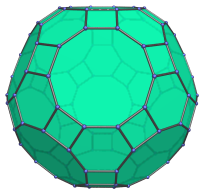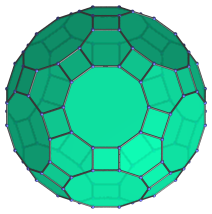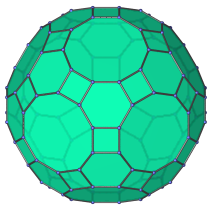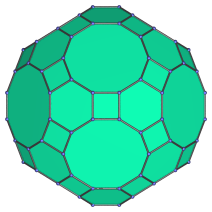# The Great Rhombicosidodecahedron

The great rhombicosidodecahedron is a 3D uniform polyhedron bounded by 62 polygons (20 hexagons, 30 squares, and 12 decagons), 180 edges, and 120 vertices. It may be constructed by radially expanding the decagonal faces of the truncated dodecahedron outwards, or equivalently, radially expanding the hexagonal faces of the truncated icosahedron, or the square faces of the rhombicosidodecahedron.The great rhombicosidodecahedron is also known as the truncated icosidodecahedron; however, this name is a misnomer, because truncating the icosidodecahedron does not yield a uniform polyhedron, only a non-uniform topological equivalent of the great rhombicosidodecahedron. The correct derivation is as described above. Note also that there is a non-convex polyhedron known as the “great rhombicosidodecahedron”, which should not be confused with this convex polyhedron.

## Projections

In order to be able to identify the great rhombicosidodecahedron in various projections of 4D objects, it is useful to know how it appears from various viewpoints. The following are some of the commonly-encountered views:

Projection Envelope DescriptionIcosagon

Parallel projection centered on a decagonal face.Icositetragon

Parallel projection centered on a hexagonal face.Dodecagon

Parallel projection centered on a square face.

## Coordinates

The Cartesian coordinates of the great rhombicosidodecahedron, centered on the origin and having edge length 2, are all permutations of coordinates and changes of sign of:

• (1, 1, 4φ+1)

together with all even permutations of coordinates and all changes of sign of:

• (1, 3+2φ, φ3)
• (2, φ4, φ2)
• 2, 2φ2, 3φ)
• (2φ, 2+φ, 1+3φ)

where φ=(1+√5)/2 is the Golden Ratio.

## Occurrences

The great rhombicosidodecahedron occurs as cells in the following 4D uniform polytopes:

Last updated 11 Jan 2023.